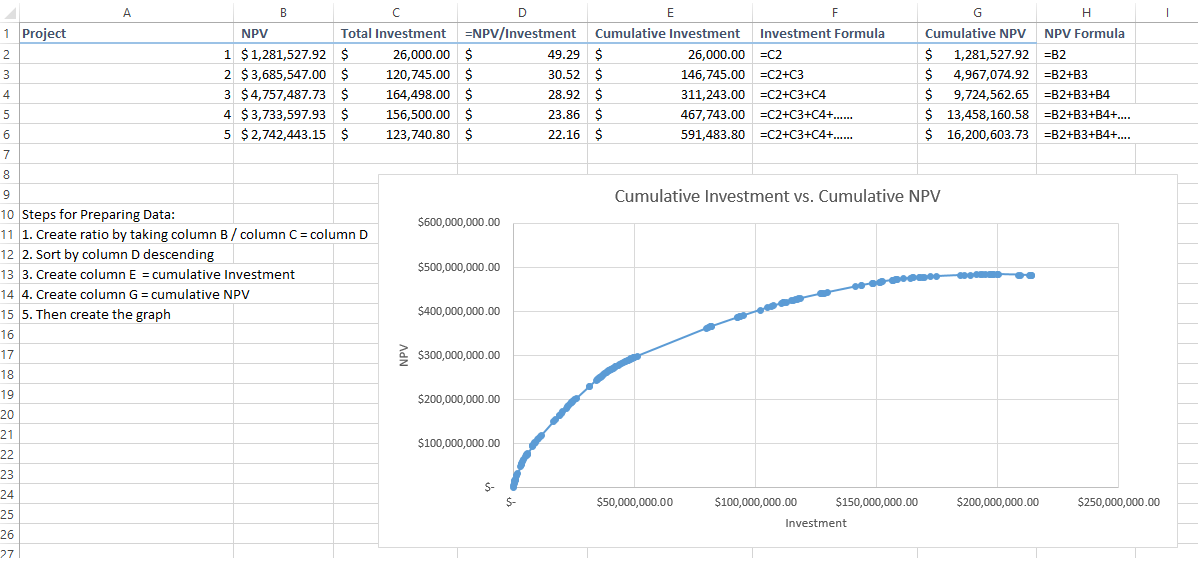# Qlik Sense App Development

Discussion board where members can learn more about Qlik Sense App Development and Usage.

Announcements
Qlik® Product Spotlight: Discover what’s possible. Get more from our products.
See for yourself. Register today.Not applicable

## Creating Cumulative Data Table for Specific Graph

Hello all:

I have a graph that needs to be created but before the graph can be created the underlying data must be manipulated in a specific order.  I have a table with projects id (Col A) , NPV (Col B), Investment (Col C), Ratio (NPV/Invest - Col D), Cumulative Invest (Col E), Cumulative NPV (Col G).  Columns F & H are just in the below file for reference as to how I need the formulas to work for columns D & E.  Columns D, E, & G are calculated columns and the only information I'm given (assume) are Project ID, NPV, and Investment.  The order that the data needs to be manipulated is:

 1. Create ratio by taking column B / column C = column D 2. Sort by column D descending 3. Create column E  = cumulative Investment (Essentially starting with row 1 and adds each subsequent row (i.e. row 1 = Invest1, row 2 = Invest1+Invest2.....) 4. Create column G = cumulative NPV (Essentially starting with row 1 and adds each subsequent row (i.e. row 1 = NPV1, row 2 = NPV1+NPV2.....) 5. Then create the graph (see below)The main issue I'm running into is the actions that need to be taken and the expression for creating the cumulative columns where row2 is equal to ColB,Row1 + ColB,Row2 and only after the data has been "ranked"/sorted by column D.  Any thoughts or suggestions would be appreciated.

Community Browser